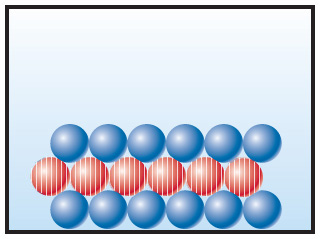# Problem: The following diagramrepresents an ionic compound in which the red spheres (six spheres with vertical stripes in the middle row) represent cations and blue spheres (12 spheres with six in the top row and six in the bottom row) represent anions.Which of the following formulas is consistent with the drawing: KBr, K2SO4, Ca (NO3) 2, Fe2 (SO4) 3?

###### FREE Expert Solution

The red spheres represent cations and the blue spheres represent anions.

Now if we have 6 red spheres to 12 blues spheres this means that we can express the ratio of the cation to anions as 6:12.###### Problem Details

The following diagramrepresents an ionic compound in which the red spheres (six spheres with vertical stripes in the middle row) represent cations and blue spheres (12 spheres with six in the top row and six in the bottom row) represent anions.

Which of the following formulas is consistent with the drawing: KBr, K2SO4, Ca (NO3) 2, Fe2 (SO4) 3?